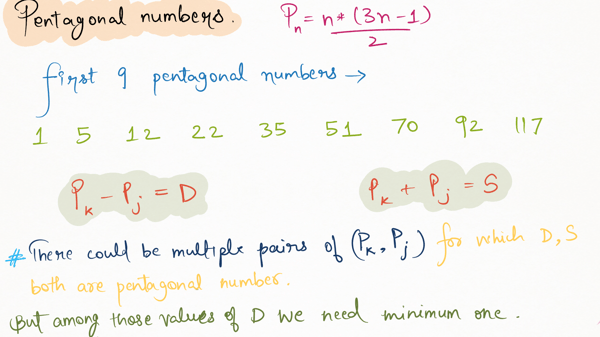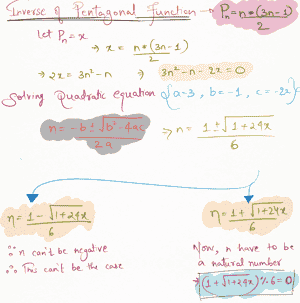Related Tags

pentagonal numbers
project euler
project euler 44
solution
communitycreator

# Project Euler 44: Pentagonal numbers

Armaan Nougai

### Problem statement

The nth term of the pentagonal sequence is defined as:

 Pn = n*(3*n - 1)/2


Find a pair of two pentagonal numbers Pj and Pk, such that:

 Pk + Pj = S is a pentagonal
number
Pk - Pj = D is a pentagonal
number and is minimum too.


What is the value of D?Question analysis

### Solution approach

We will run two loops:

• an outer loop over pentagonal sequence for the value of P(k).
• an inner loop over pentagonal sequence less than P(k) for P(j).

Then, we will calculate and check if D and S are pentagonal. To check this, we will inverse the pentagonal function.

The moment we get a pair for which D and S are pentagonal, we’ll break the loop and return D. This is because the first pentagonal D is also the minimum one.Inverse of pentagonal function

### Solution code

"""
Coded by - Armaan Nougai
"""

from math import sqrt

def is_pentagonal(n): return (1+sqrt(1+24*n))%6==0

i=0
while True:
i+=1
k = i*(3*i-1)//2
for v in range(1,i):
j = v*(3*v-1)//2
if is_pentagonal(k-j) and is_pentagonal(k+j) :
print(k-j)
break
else:
continue
break

Why is the first value of D (i.e., 5482660) the minimum possible value of D?

On analyzing the pentagonal sequence:

1. P(2)-P(1) = 4

2. P(3)-P(2) = 7

3. P(4)-P(3) = 10

##### Generalizing:
P(n)-P(n-1) < P(n+1)-P(n) < P(n+2)-P(n+1) ...


This means the difference between adjacent terms is increasing.

And also, for the nth term, the minimum D, even without the condition, will be:

P(n)-P(n-1)


Now, after getting the first value of D, we will continue the search for the lesser value of D until we find an adjacent pair that satisfies the condition and whose D is greater than the first value of D.

This is because, after this point, the D of every pair will be greater than the first value of D.

RELATED TAGS

pentagonal numbers
project euler
project euler 44
solution
communitycreator

CONTRIBUTOR

Armaan Nougai
RELATED COURSES

View all Courses

Keep Exploring

Learn in-demand tech skills in half the time